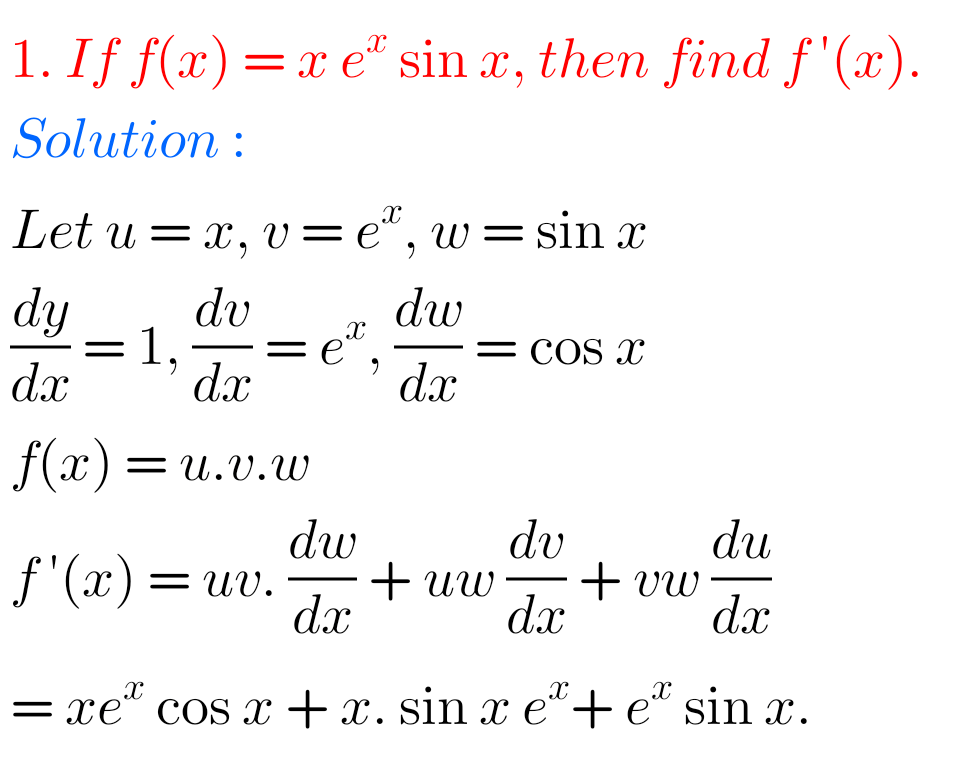# Intermediate Maths Solutions for Differentiation exercise 9(b)

Differentiation exercise 9(b) textbook solutions Inter Maths 1B are given.

Study the textbook lesson Differentiation very well.

Observe the example problems and solutions given in the textbook.

Observe the given below solutions and try them in your own method.

You can also see

Inter Maths 1A textbook solutions

Inter Maths 1B textbook solutions

Inter Maths IIA textbook solutions

Inter Maths IIB textbook solutions

Differentiation

Exercise 9(a)

Exercise 9(b)

Exercise 9(c)

Exercise 9(d)

M

## Differentiation exercise 9(b) solutions Inter Maths 1B

Chapter 9 – Differentiation

Exercise 9(b)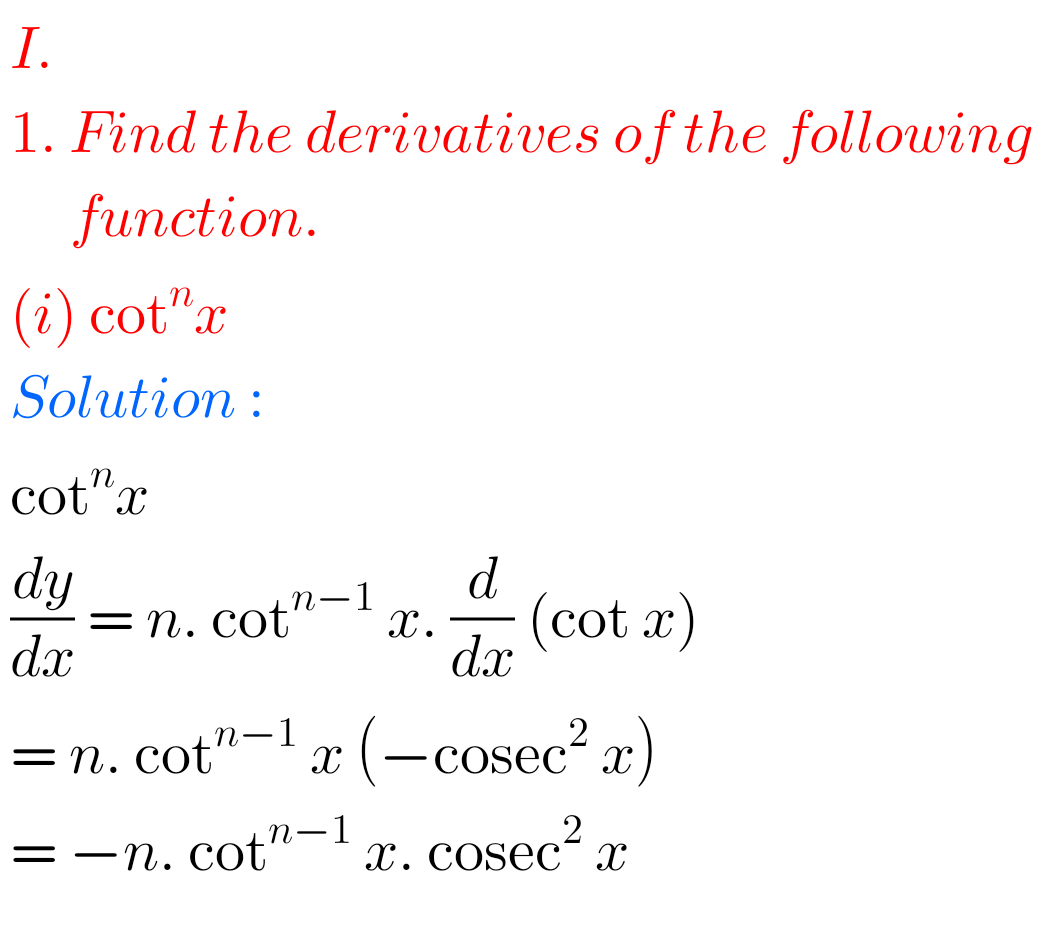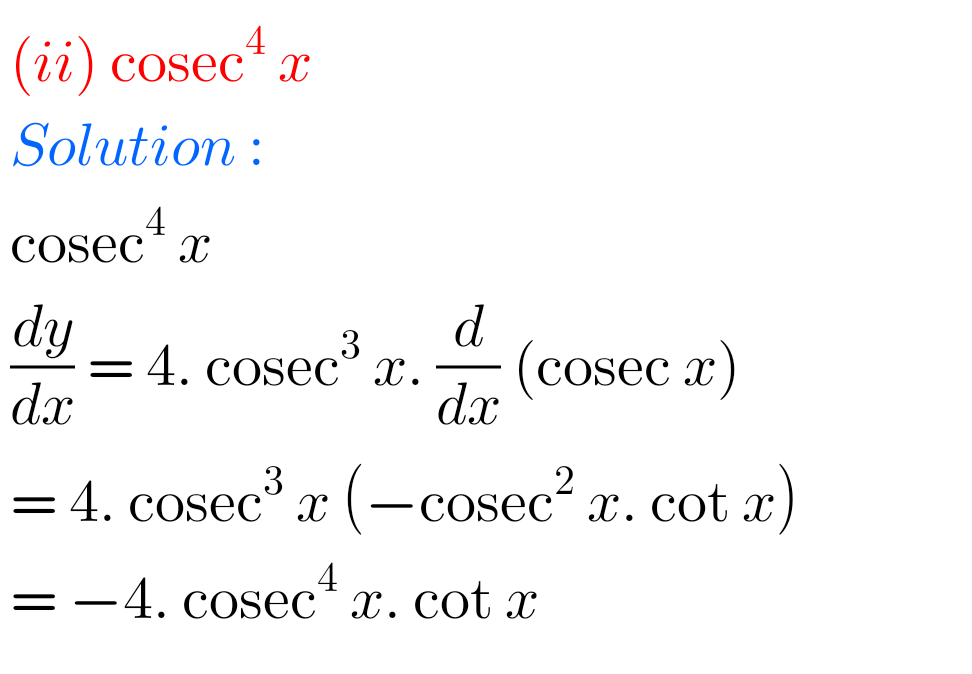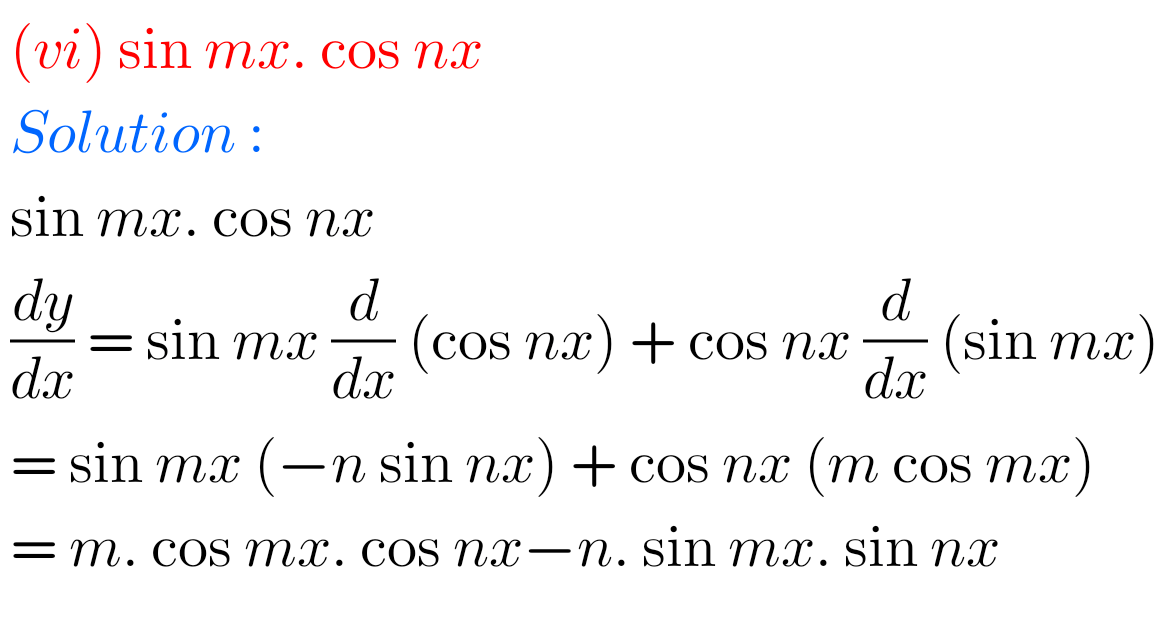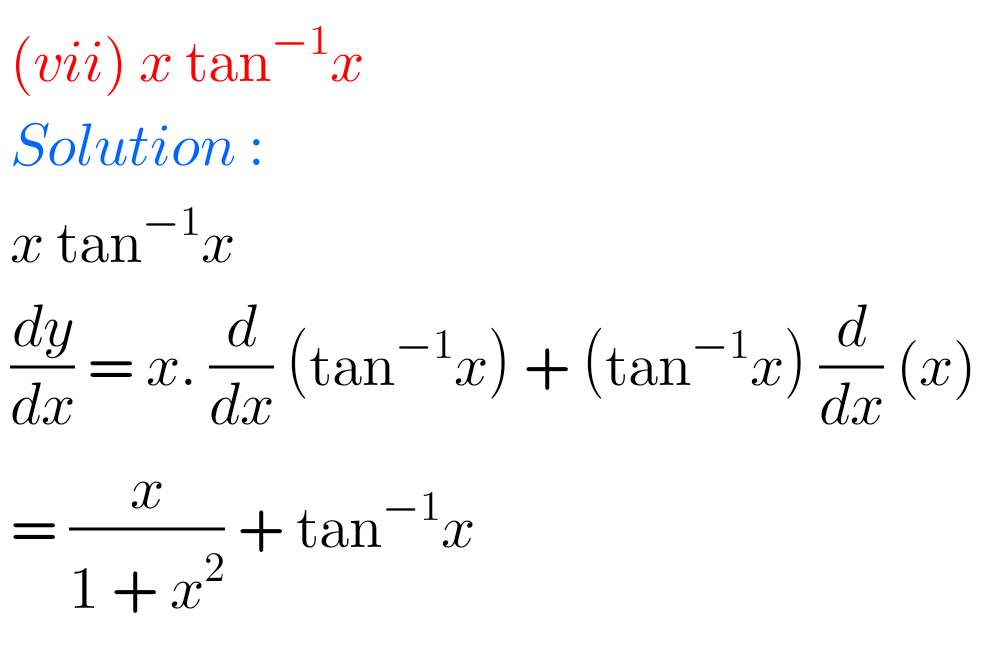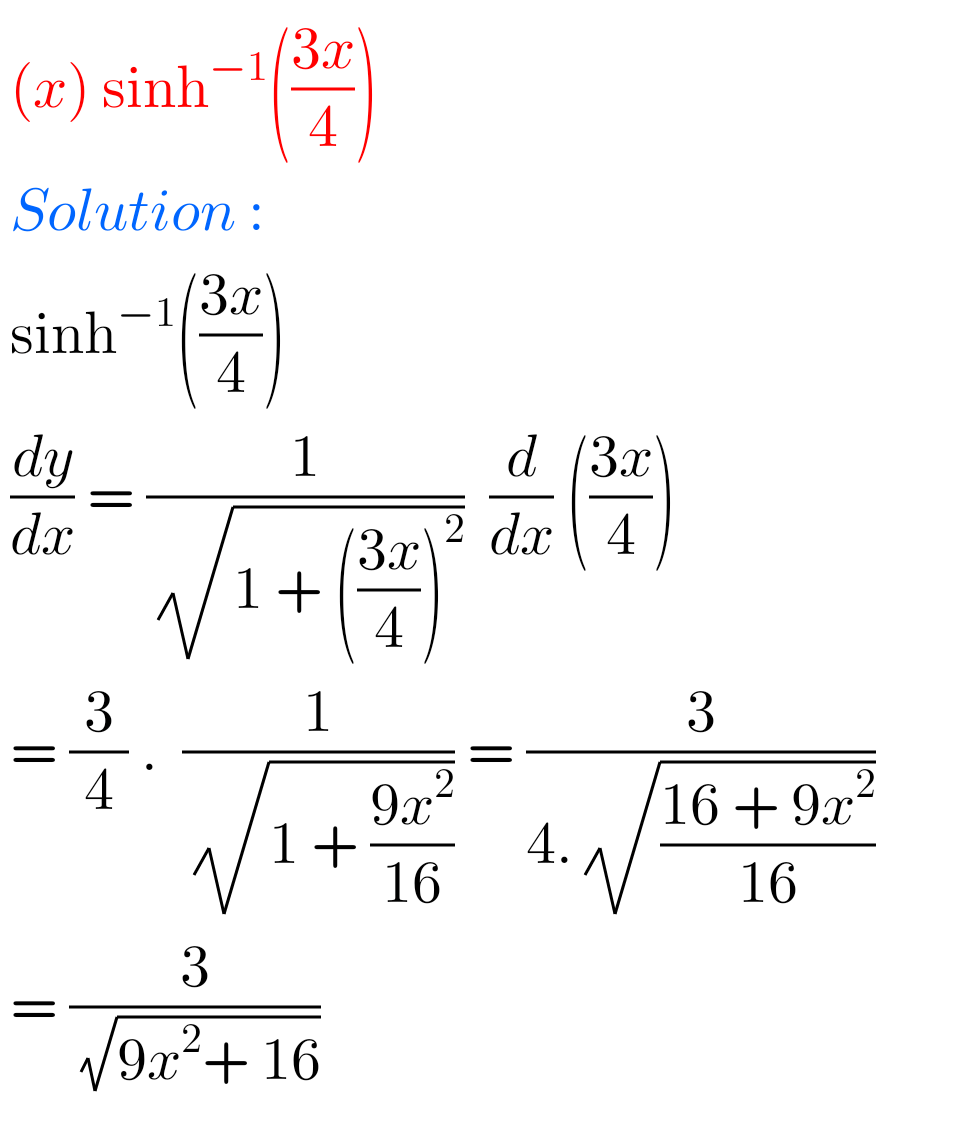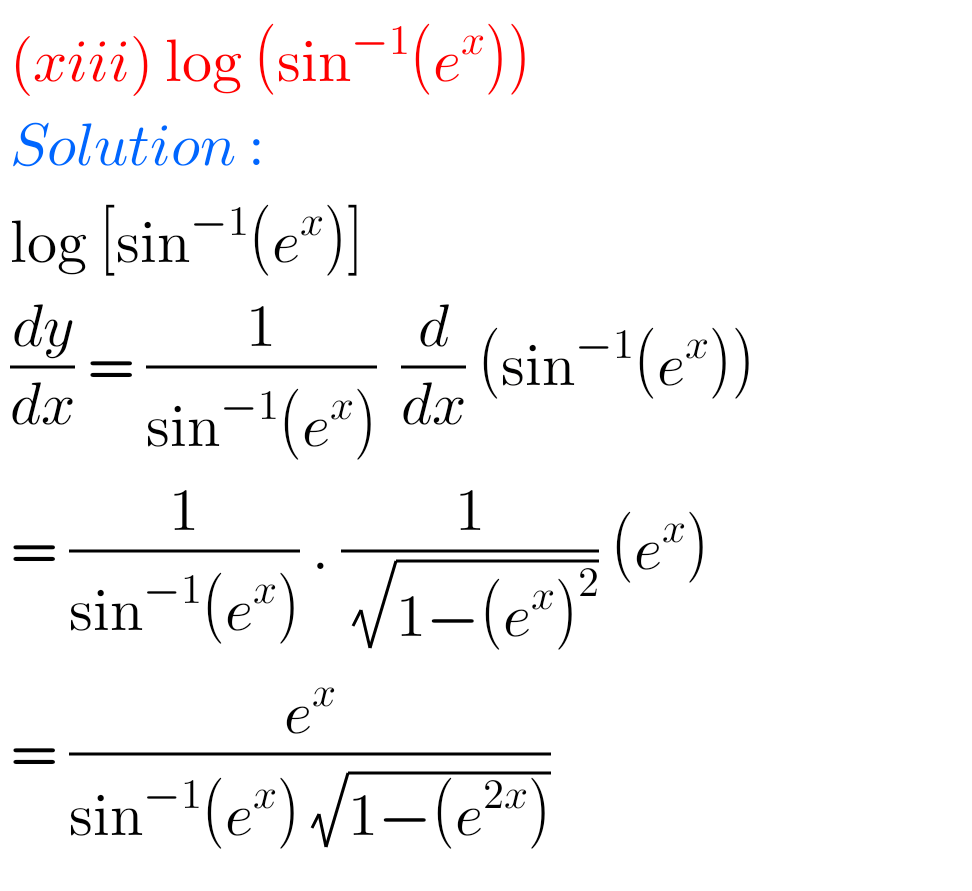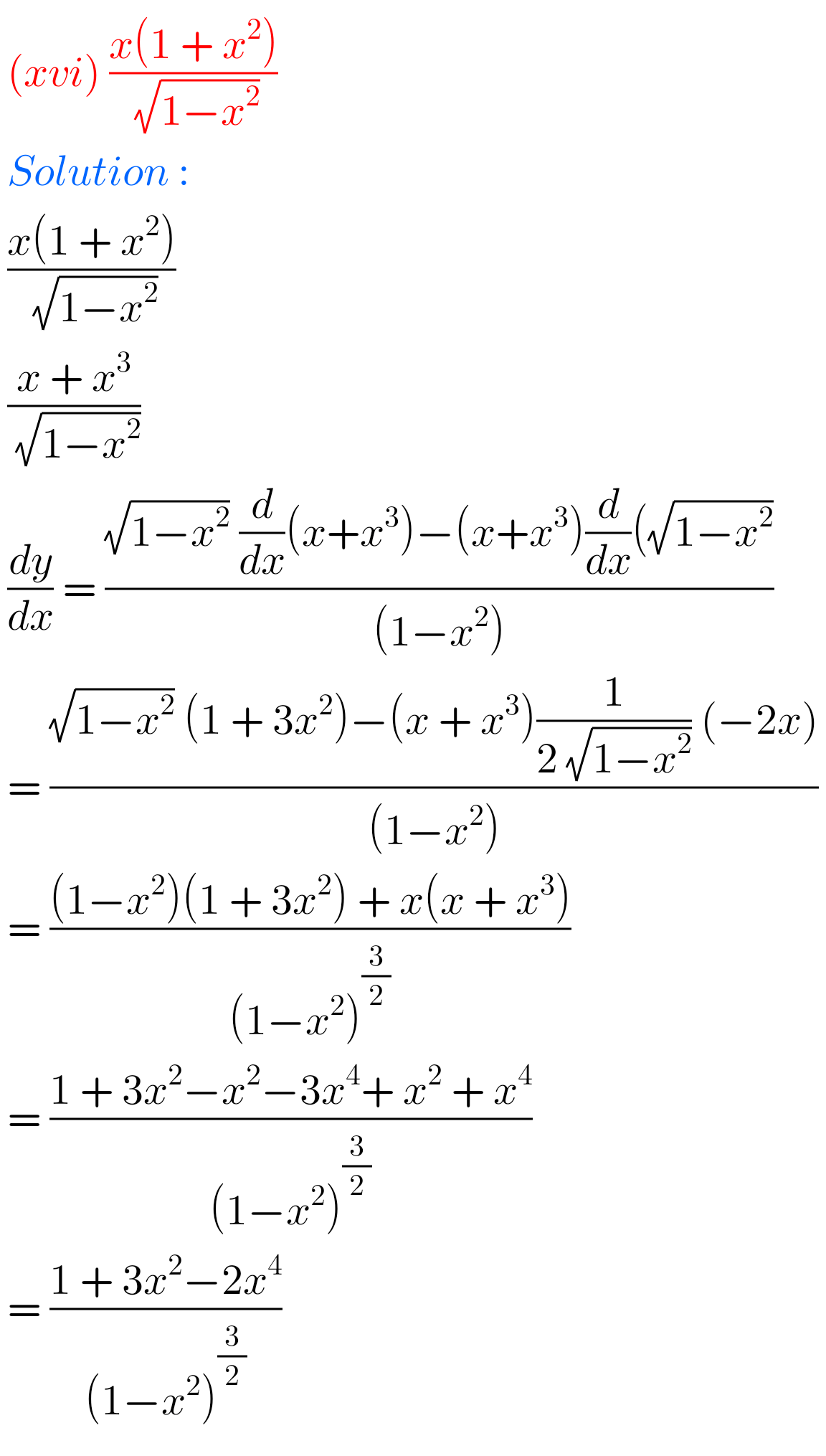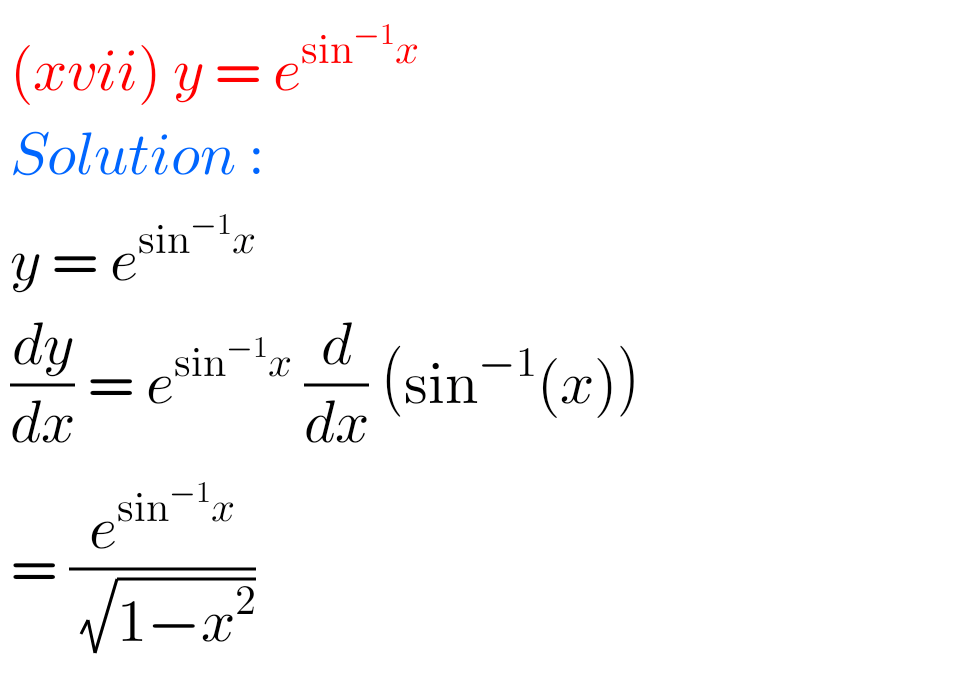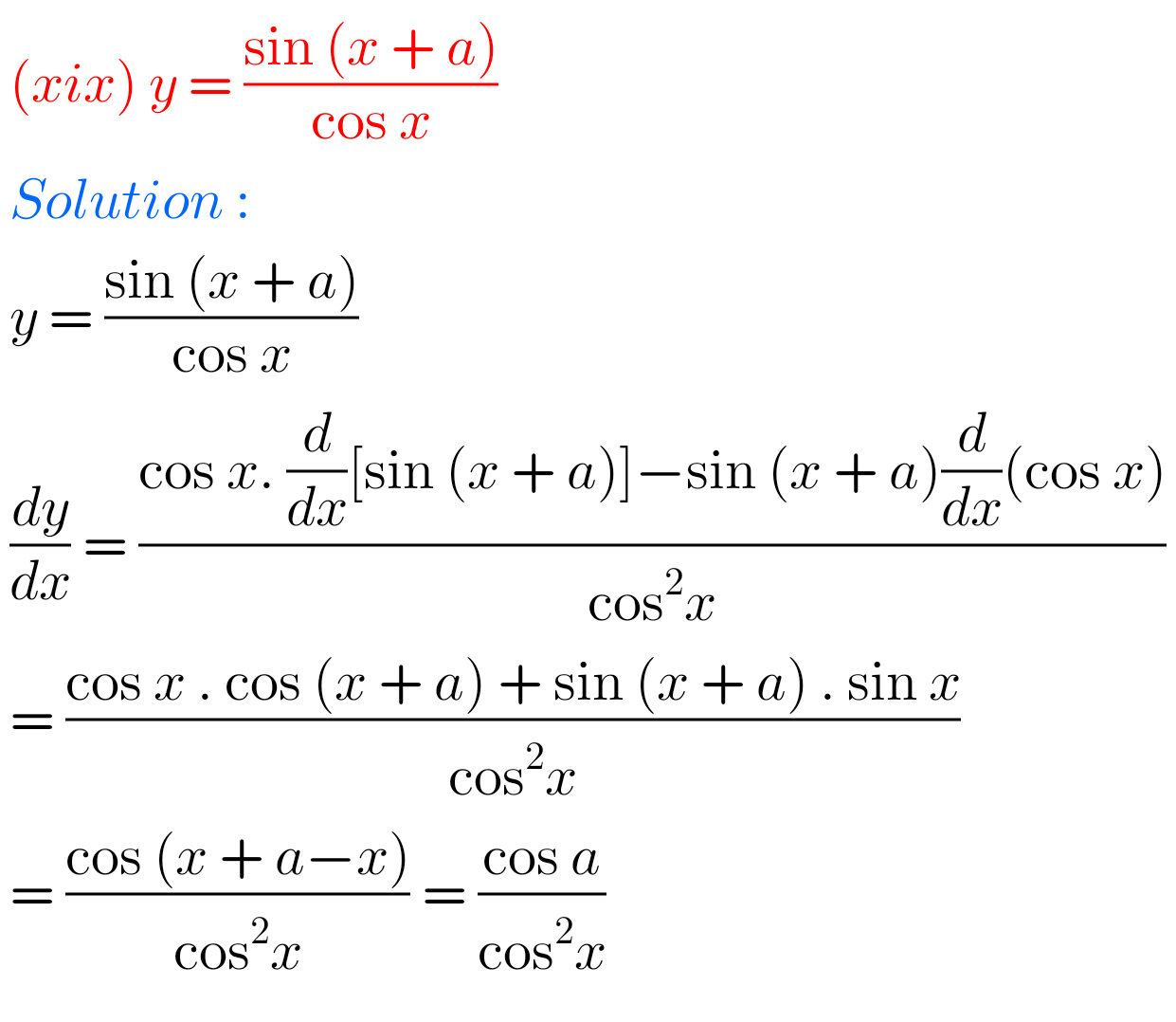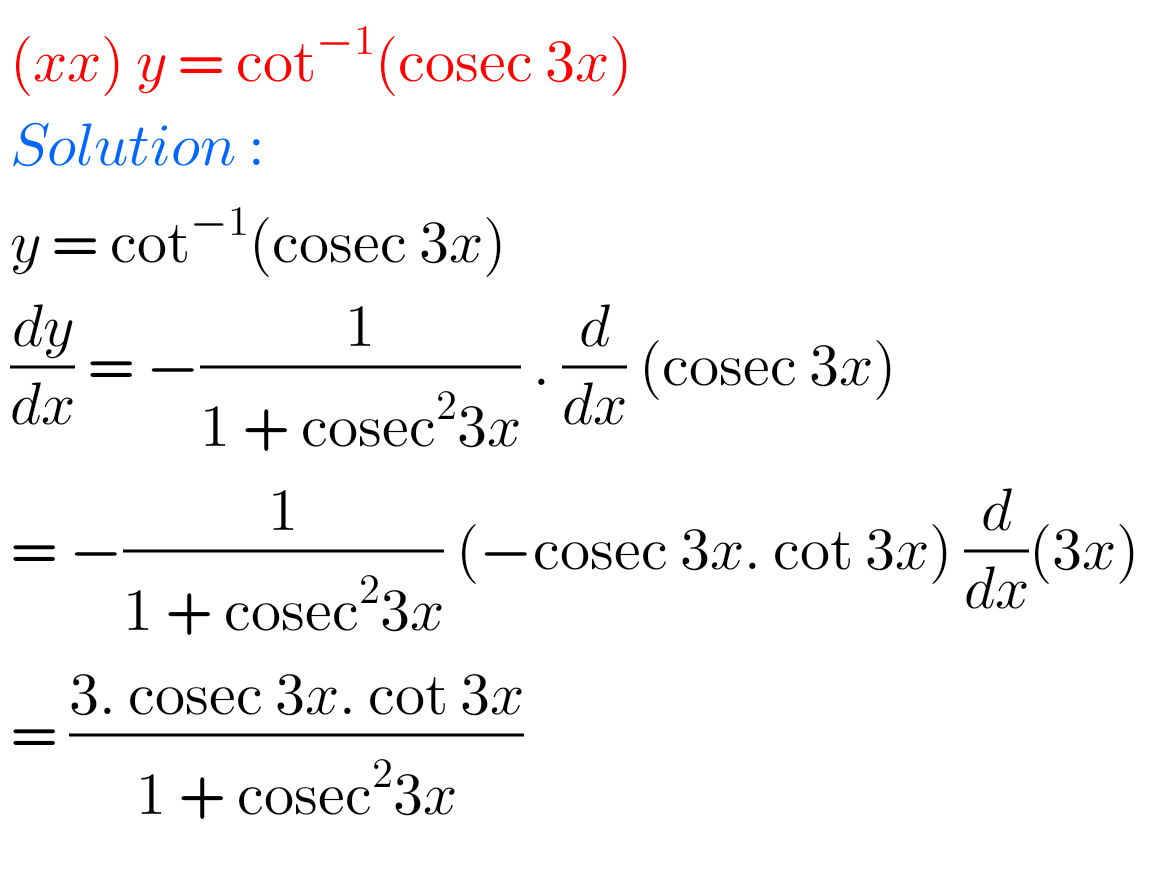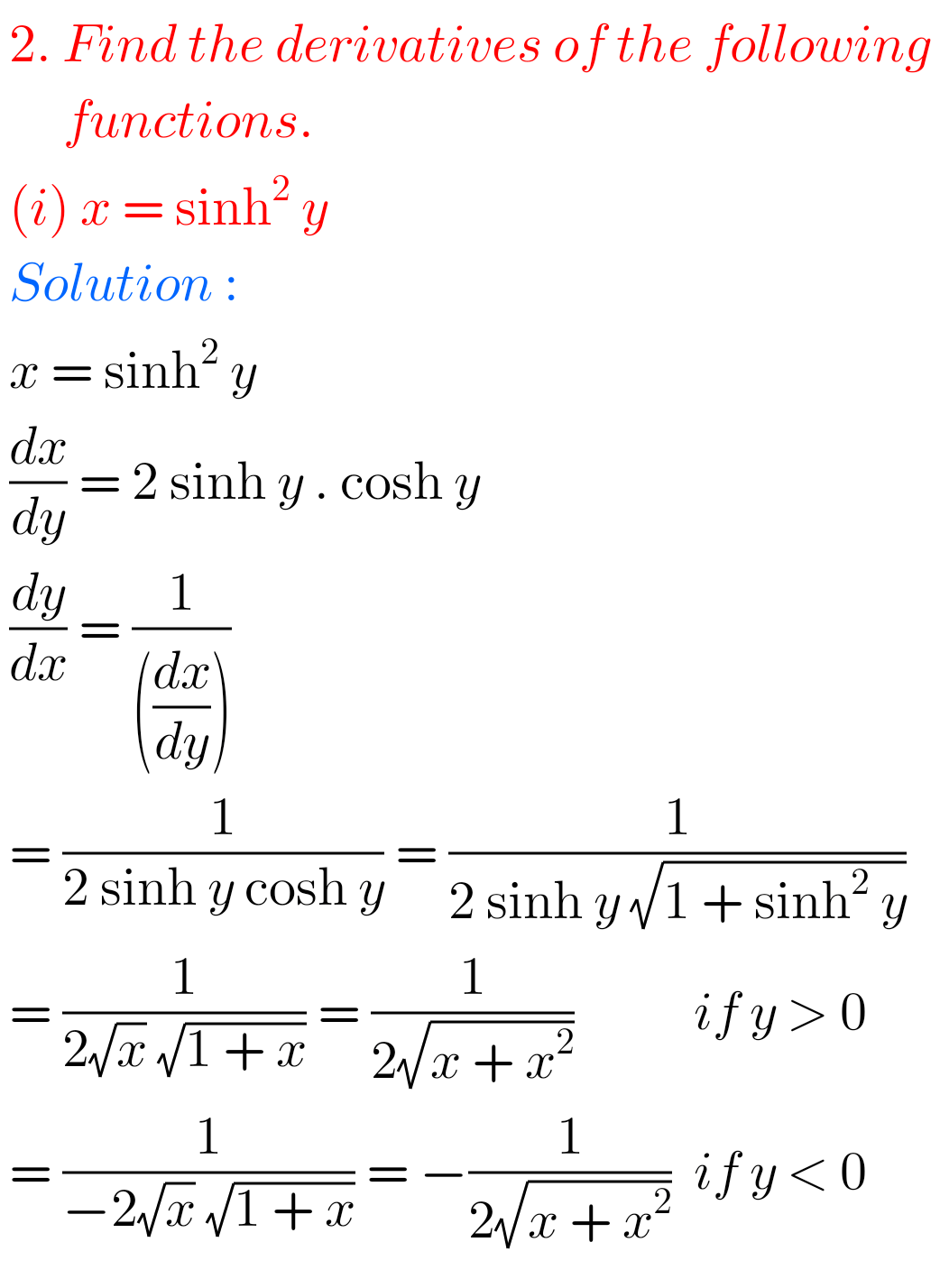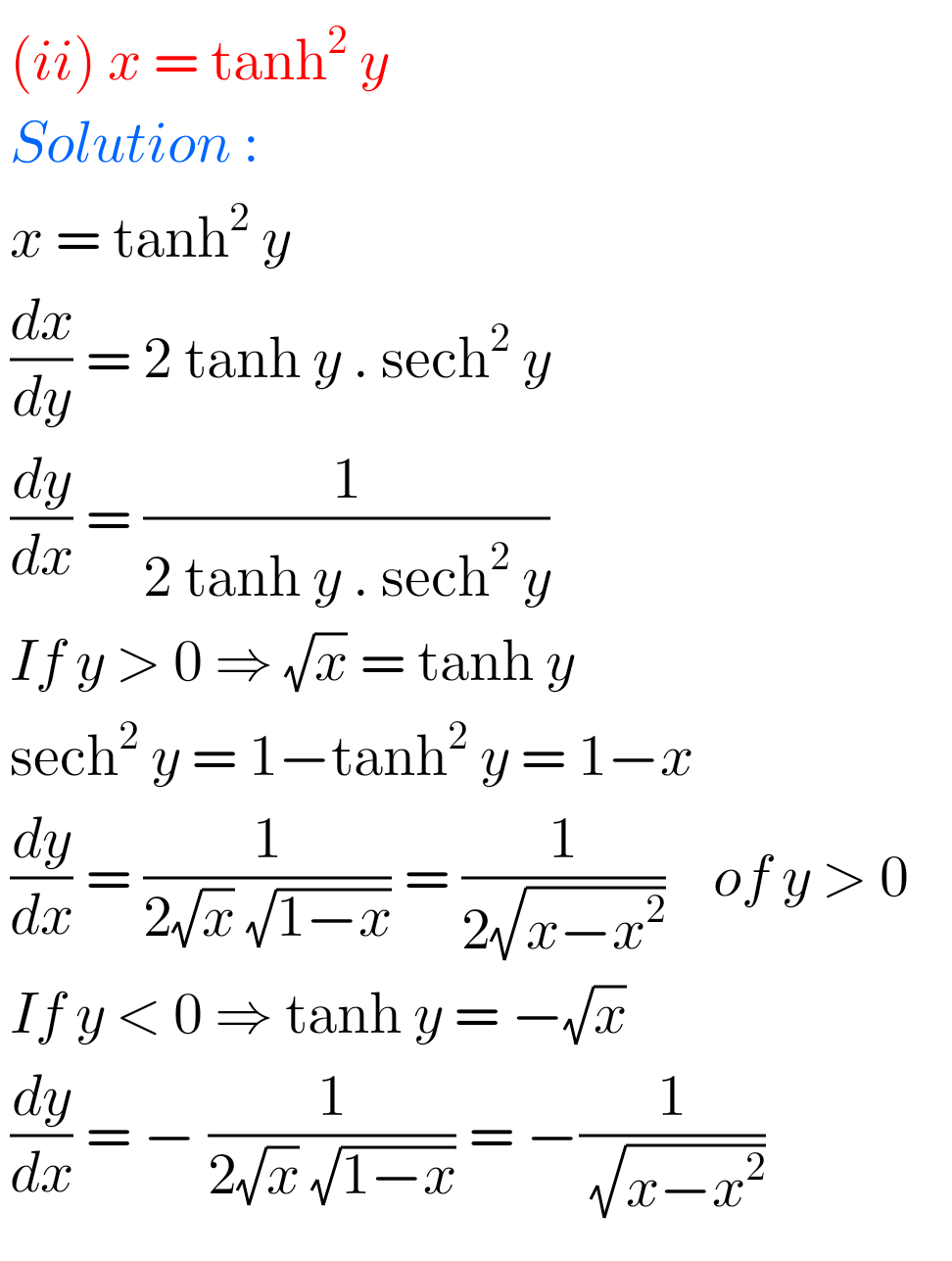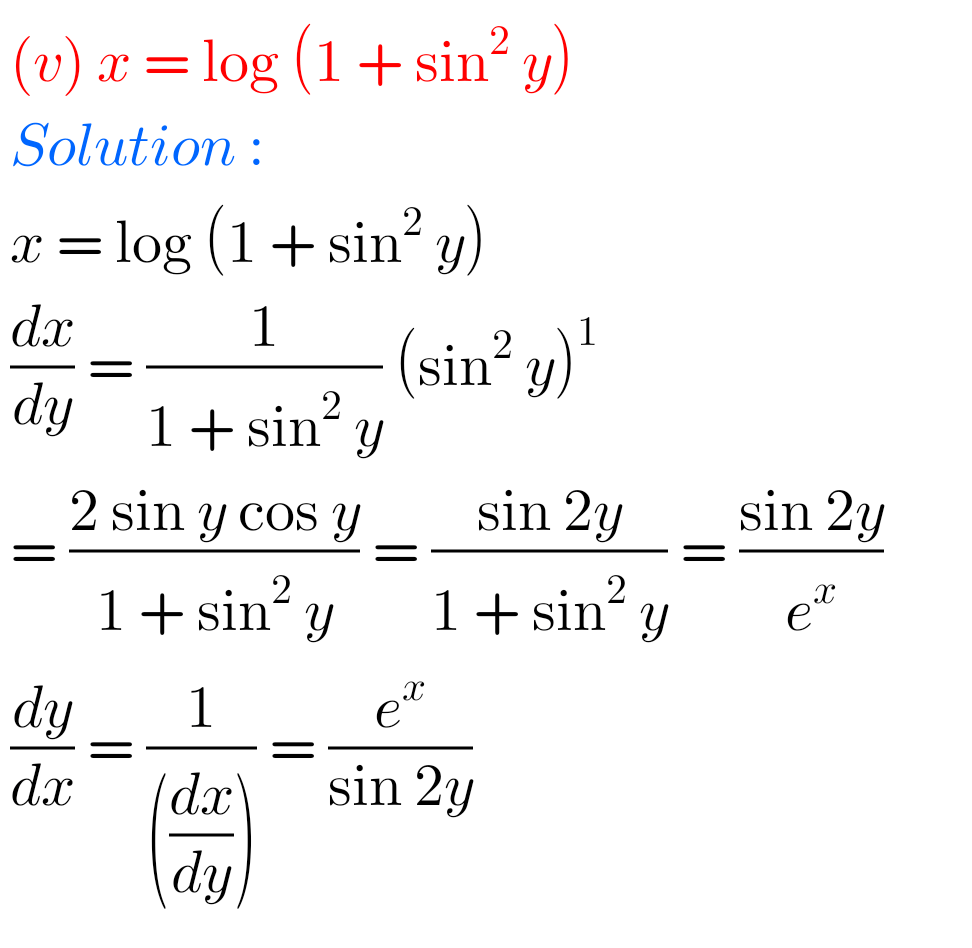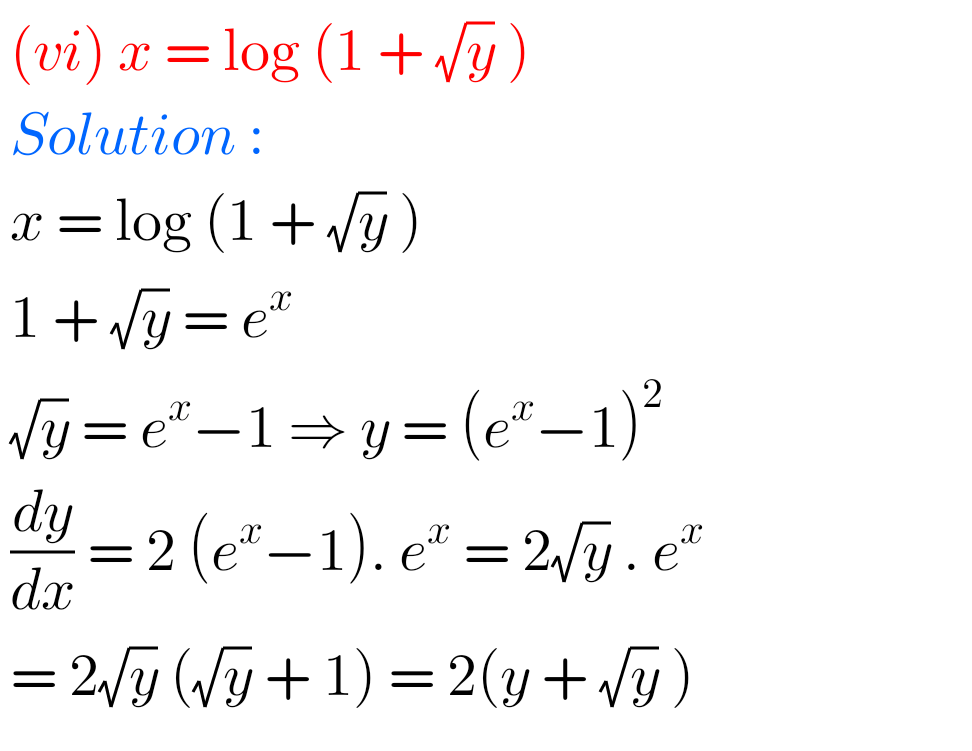## Solutions for Maths Inter Differentiation Exercise 9(b)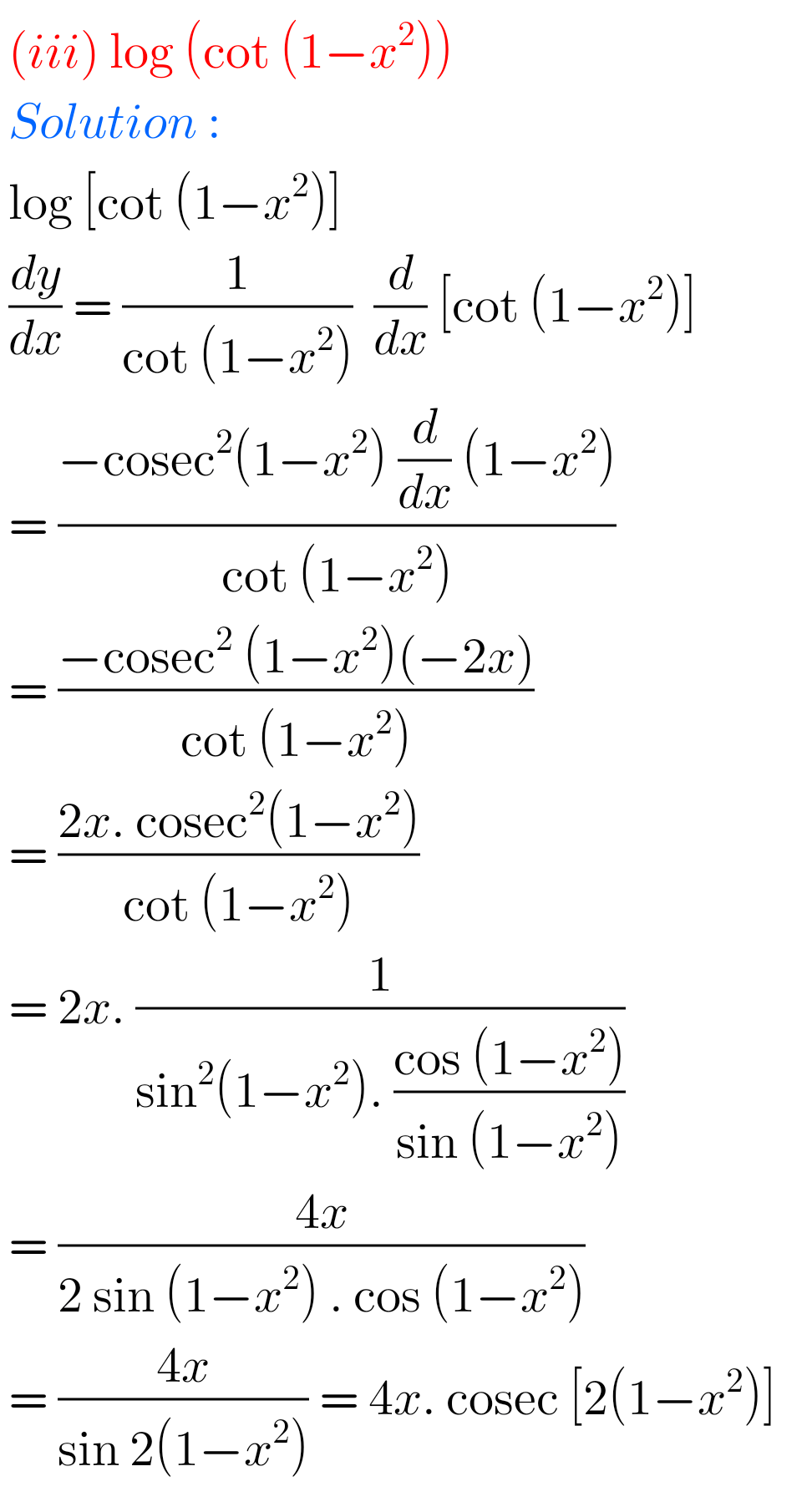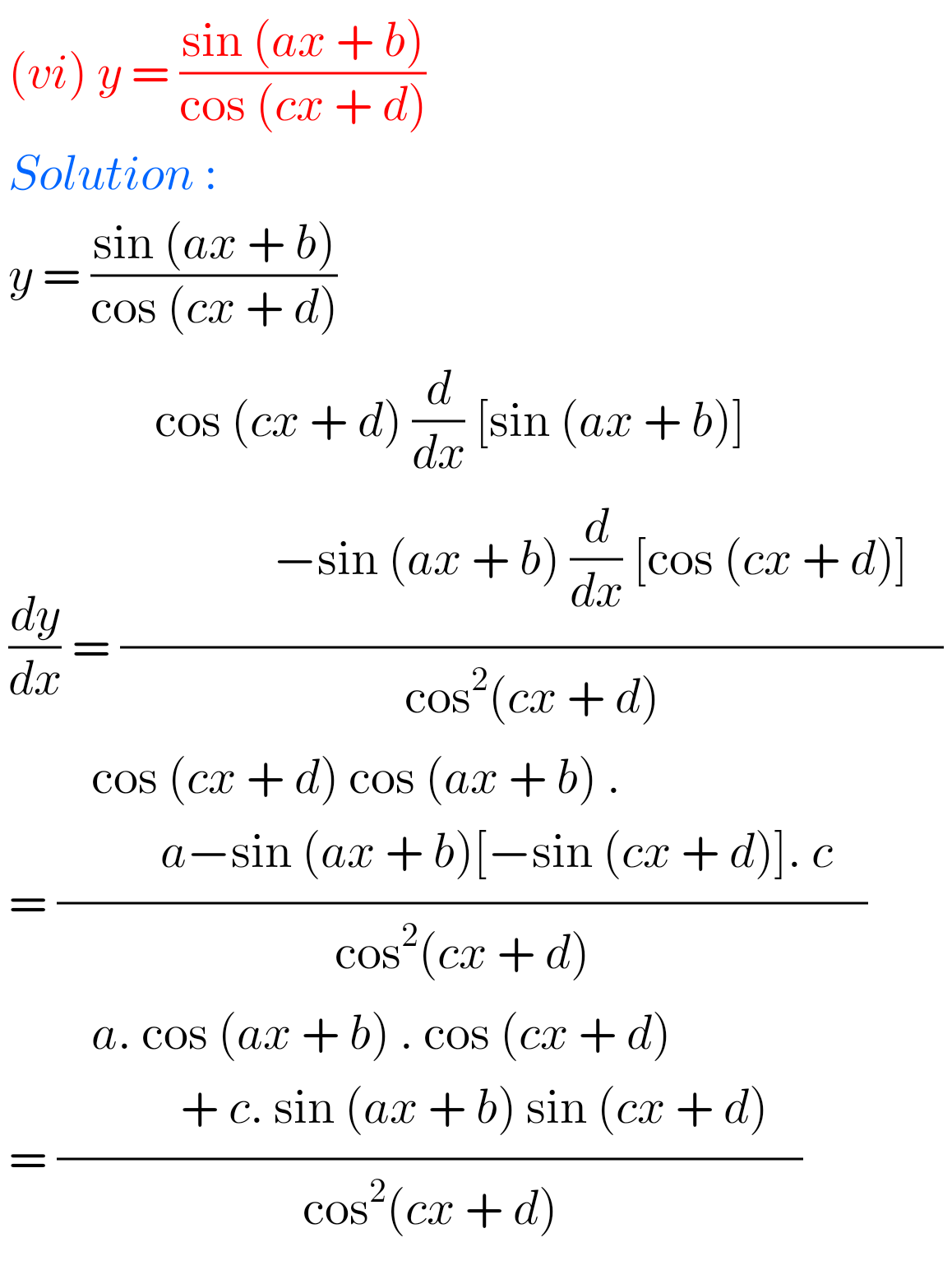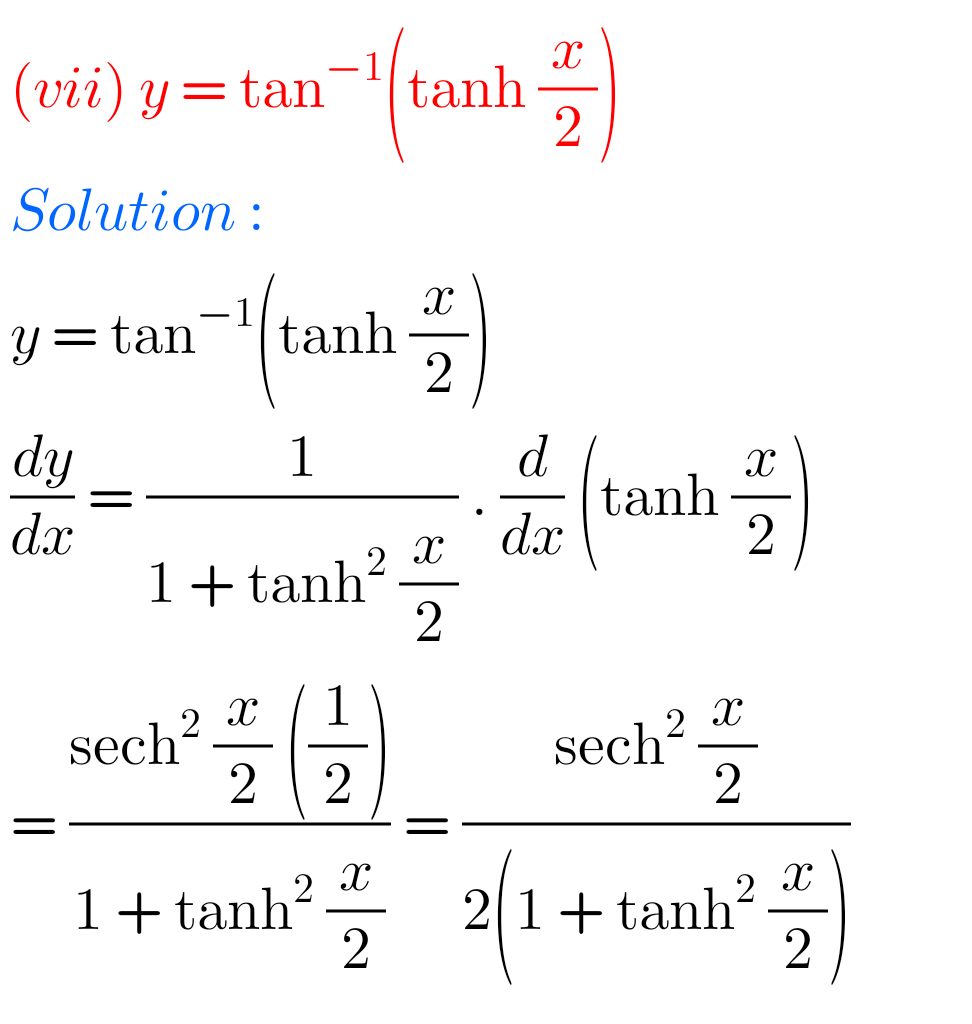## Exercise 9(b) Differentiation Inter Maths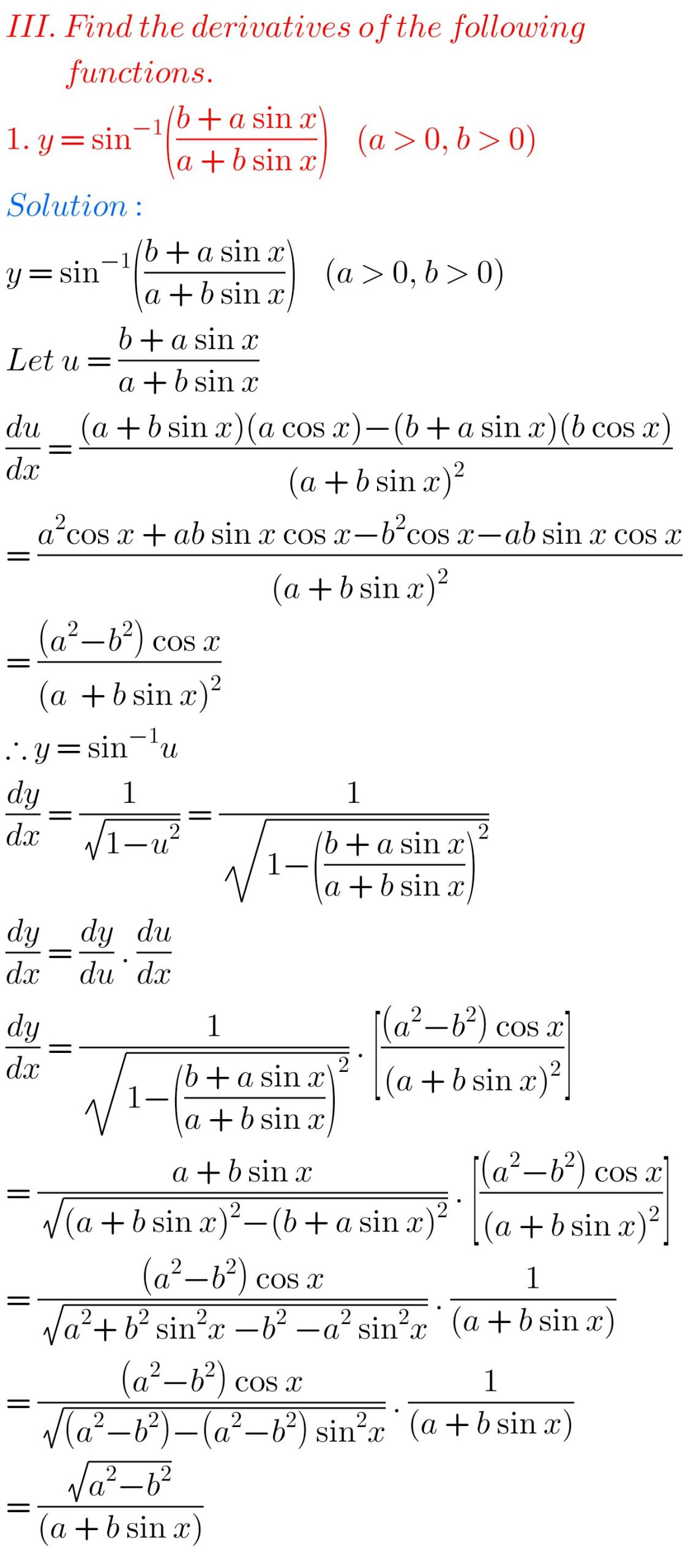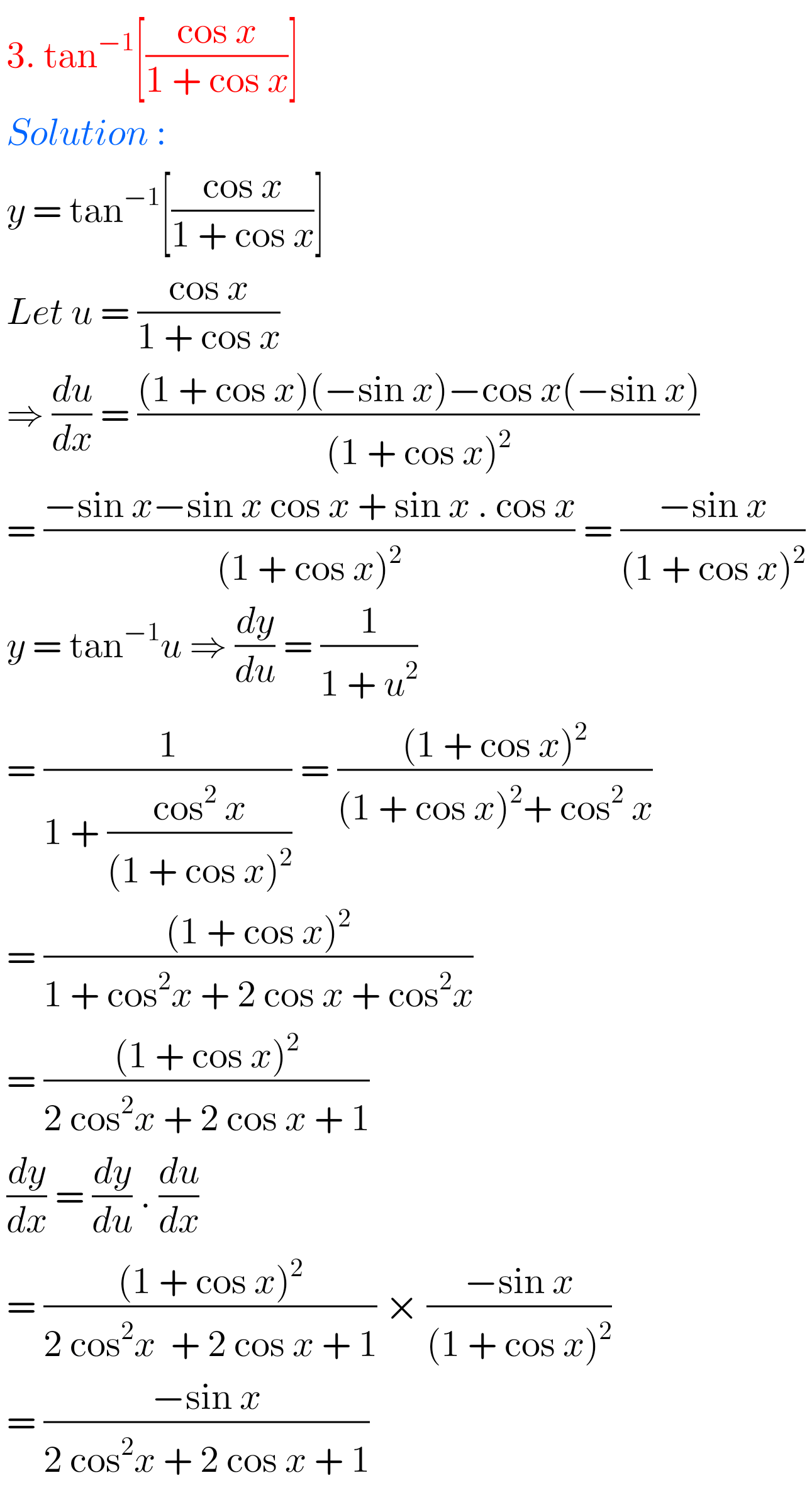Note : Observe the solutions and try them in your own method.